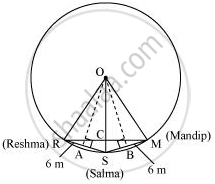Share

# Three girls Reshma, Salma and Mandip are playing a game by standing on a circle of radius 5m drawn in a park. Reshma throws a ball to Salma, Salma to Mandip, Mandip to Reshma. - CBSE Class 9 - Mathematics

ConceptEqual Chords and Their Distances from the Centre

#### Question

Three girls Reshma, Salma and Mandip are playing a game by standing on a circle of radius 5m drawn in a park. Reshma throws a ball to Salma, Salma to Mandip, Mandip to Reshma. If the distance between Reshma and Salma and between Salma and Mandip is 6m each, what is the distance between Reshma and Mandip?

#### Solution

Draw perpendiculars OA and OB on RS and SM respectively.AR = AS = 6/2 = 3m

OR = OS = OM = 5 m. (Radii of the circle)

In ΔOAR,

OA2 + AR2 = OR2

OA2 + (3 m)2 = (5 m)2

OA2 = (25 − 9) m2 = 16 m2

OA = 4 m

ORSM will be a kite (OR = OM and RS = SM). We know that the diagonals of a kite are perpendicular and the diagonal common to both the isosceles triangles is bisected by another diagonal.

∴∠RCS will be of 90° and RC = CM

Area of ΔORS = 1/2 x OA x RS

1/2 x RC x OS = 1/2 x 4 x 6

Rc x 5 = 24

RC = 4.8

RM = 2RC = 2(4.8) = 9.6

Therefore, the distance between Reshma and Mandip is 9.6 m.

Is there an error in this question or solution?

#### APPEARS IN

NCERT Solution for Mathematics Class 9 (2018 to Current)
Chapter 10: Circles
Ex. 10.40 | Q: 5 | Page no. 179

#### Video TutorialsVIEW ALL 

Solution Three girls Reshma, Salma and Mandip are playing a game by standing on a circle of radius 5m drawn in a park. Reshma throws a ball to Salma, Salma to Mandip, Mandip to Reshma. Concept: Equal Chords and Their Distances from the Centre.
S